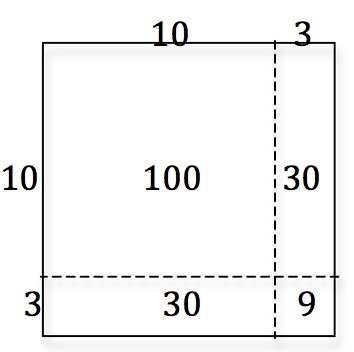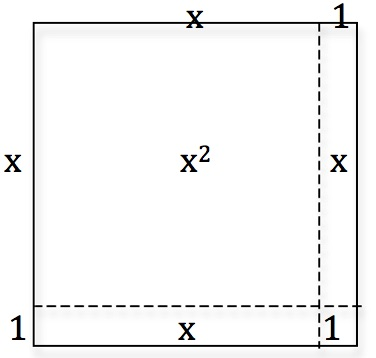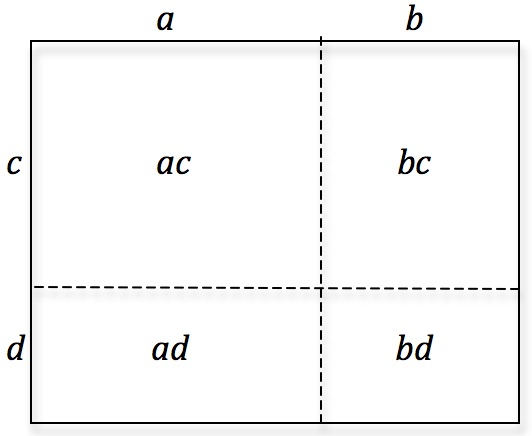Explain how the diagrams show how to work out 13 times 13 and (x+1) times (x+1).

Draw similar diagrams for 15 times 15 and (x+2) times (x+2).

Draw your own diagrams and use the same method to find the algebraic expressions for $(x + 3)^2$ and $(2x + 5)^2$ and work out the expansion of $(a + b)(c + d)$.Tagged with: